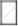# Fill in the Boxes with the Correct Symbol Out of >, <, and =1by(-3) and (-1)By4 - Mathematics

Sum

Fill in the boxes with the correct symbol out of >, <, and =

1/(-3)(-1)/4

#### Solution

(- 1)/3 = (-1 xx 4)/(3 xx 4) = (- 4)/12

= (- 1)/4 = (-1 xx 3)/(4 xx 3) = (- 3)/12

Thus, (- 1)/3 [<] (- 1)/4

Concept: Rational Numbers Between Two Rational Numbers
Is there an error in this question or solution?

#### APPEARS IN

NCERT Class 7 Maths
Chapter 9 Rational Numbers
Exercise 9.1 | Q 8.5 | Page 183